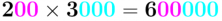Smartick is a fun way to learn math!Aug15

# Multiply Numbers Ending with Zero(s)

Hello everyone! This week I’m bringing you a post about how to multiply by a number ending with zeros. It’s super easy!

In order to multiply by a number ending in zeros, we first need to multiply the number as if it didn’t have zeros, and after, we just add all of the zeros the number had to the final answer.

For example:

4,000 × 6

We ignore the zeros that 4000 had and multiply the two numbers,

4 × 6=24

Now, we just add the 3 zeros that the number 4000 had to get:

24,000

We’re going to take a look at another example of how to multiply a number that ends in zeros, but this time, both numbers are ending with zeros:

50 × 8,000

Now, both the 50 and the 8000 have zeros. We’ll do the same and ignore them for now and multiply the numbers.

5 × 8=40

Then, we add a zero from the 50 and 3 zeros from the 8000, in total we’ll add 4 zeros.

400,000

To wrap up, I’ll leave you guys with another example:

200 x 3,000

2 x 3 = 6. We have to add 2 zeros from the 200 and 3 zeros from the 3000. In total, we’ll have to add 5 zeros.If it was helpful to you and you learned how to multiply by a number that’s ending with zeros, don’t hesitate to share it with your friends so that they can learn, too. Thanks a lot for following Smartick’s blog and we’ll see you next time!

If you want to practice multiplying with numbers that end with zeros and other elementary math topics, register on Smartick and try it for free!